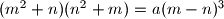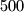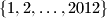### IMO Shortlist 2012 problem N4

Kvaliteta:
Avg: 0,0
Težina:
Avg: 7,0
An integer$a$ is called friendly if the equation$(m^2+n)(n^2+m)=a(m-n)^3$ has a solution over the positive integers.
a) Prove that there are at least$500$ friendly integers in the set$\{ 1,2,\ldots ,2012\}$.
b) Decide whether$a=2$ is friendly.
Izvor: Međunarodna matematička olimpijada, shortlist 2012